There are all sorts of resonances around us, in the world, in our culture, and in our technology. A tidal resonance causes the 55 foot tides in the Bay of Fundy. Mechanical and acoustical resonances and their control are at the center of practically every musical instrument that ever existed. Even our voices and speech are based on controlling the resonances in our throat and mouth. Technology is also a heavy user of resonance. All clocks, radios, televisions, and gps navigating systems use electronic resonators at their very core. Doctors use magnetic resonance imaging or MRI to sense the resonances in atomic nuclei to map the insides of their patients. In spite of the great diversity of resonators, they all share many common properties. In this blog, we will delve into their various aspects. It is hoped that this will serve both the students and professionals who would like to understand more about resonators. I hope all will enjoy the animations.

Origins of Newton's laws of motion

History of mechanical clocks with animations
Understanding a mechanical clock with animations
includes pendulum, balance wheel, and quartz clocks

## Tuesday, October 17, 2017

### Games with arrays of wave emitters

Contents of this posting

## Games with arrays of wave emitters

Assumptions of the emitter method
• All surfaces, reflectors and absorbers are replaced by linear arrays of emitters.
• Emitted waves are free to propagate in the whole space.
• Emitters only emit waves. Other than adding to the wave field, they do not affect the already emitted waves in any way. They do not reflect or absorb waves.
• Reflection and absorption are accomplished by emitters adding a wave that destructively adds (i.e. subtracts) from the existing wave field.

One approach to solving a problem of waves in the presence of reflecting and absorbing walls is to model the problem with arrays of emitters. That is, replace all walls, reflectors and absorbers with emitters, nothing but emitters, and let the waves be free to propagate from these emitters without any objects in the space. In this article we examine the possibilities in algebra and images of the wave patterns of various emitter arrays in two dimensions.

One of the motivations for using this method is the number of variables involved. Conventional computer modeling of waves in the 2D world divides up the space into a 2D array of cells, each with variables to be calculated. For a two dimensional problem, the emitter method involves linear arrays of emitters and no 2D arrays of variables. It is therefore computationally cheaper.

In 2D differential equation methods, a user needs to add features to absorb radiation that should disappear into space. In emitter methods, this issue does not occur.

Emitter methods are most appropriate for systems that are mostly made up of a simple wave medium.

### Single emitters - types

#### A monopole emitter

The equation for the 2D wave field around a monopole emitter located at the origin is:

where H0(1) is a Hankel function of order 0 and of the first kind. The Hankel function is a complex sum of Bessel functions of the first and second kinds with a 90 degree phase shift, i.e. H0(1) = J0 + i Y0.

The wave field is a function of the wavenumber κ = /λ, where r (the distance between the emitter and point of field measurement which equals the polar coordinate radius for an emitter at the origin), and λ which is the wavelength of the wave being emitted (measured a distance away from the emitter where the waves approximate a plane wave).

The variable Φ in (1) above is the distance varying part of the potential of the wave. We have not included the time part of the potential which is understood to be e−iωt. The actual or physical wave potential is the real part of the total complex potential (that is the real part of (1) after it is multiplied by the time part).  Φ is the velocity potential in the case of water waves. The parameter A in (1) sets the amplitude of the wave.

Below we show a video of such waves from an emitter at the origin.

 Fig. 1. Video clip of the waves being emitted by a monopole emitter at the origin and given by (1), that is the real part of (1) after it is multiplied by the implied time part. Note that these waves have equal strength in all directions. Figs. 2a and 2b (below). The left figure is a snapshot image of the wave shown in Fig. 1 and specified by Equation 1. The wave is frozen in time as if you had taken a photograph of it. The right figure below shows the amplitude of the wave in the space. Another way to think of the right figure is that it is the maximum wave experienced at any given point as the wave sweeps over that point. In two dimensions the amplitude of waves from a monopole falls off as 1/√r .#### A dipole emitter

The equation describing the wave field coming from a dipole emitter in two dimensions (2D) is as given in (2):

where H1(1) is a Hankel function of order 1 and of the first kind.

Note that in Fig. 3 below, the wave field is not uniform in all directions as it is in Figs. 1 and 2 but varies with direction as given by the cos ϕ as given by (2). This wave field is strongest in the positive and negative x directions where ϕ = 0  and ϕ = π . The wave field is zero in the positive and negative y-directions where ϕ = π/2  and ϕ = 3π/2 .

 Fig. 3. Video clip of the waves from a dipole emitter at the origin and given by (2) above. Note that these waves are strongest along the positive and negative x axis. They are zero along the y axis. Note also that the waves on the right and left sides are phased 180 degrees apart. That is, when a wave crest on the right side is red (positive), the corresponding mirror crest on the left side is blue (negative). Figs. 4a and 4b (below). The left figure is a snapshot of the wave shown in Fig. 3 and specified by Equation 2. It is as if the wave were frozen in time. The right figure is a mapping of the amplitude of the wave. In two dimensions the amplitude of waves from a dipole falls off as 1/√r .### A directional emitter

To get an emitter that sends waves mostly in one direction, we combine the two above emitters with a 90 degree phase shift as given by:

We see the results in the images Figs. 5 and 6 below.

 Fig. 5. Video clip of the fields around a directional emitter as given by (3) above. Figs. 6a and 6b. Snapshot and amplitude of a directional emitter located at the origin. Note that the waves are maximum along the positive x axis and zero along the negative x axis. Note that the origin is left of center as indicated by the axis numbering. The amplitude varies as (cos φ  + 1) . In two dimensions the amplitude of waves from a directional emitter falls off as 1/√r . Note that this (cos φ  + 1)  dependence is consistent with the Huygens-Kirchhoff diffraction formula [Elmore and Heald, physics of waves, p.331]. More on this below.### Arrays of emittersLocation of the linear array of emitters used to make field mappings in Figs. 7, 8 and9.

The Huygens-Kirchhoff diffraction formula is based on the idea that the wavefield in any space can be calculated based solely on the wave entering the space to power the wavefield there. The Huygens-Kirchhoff formula is basically just setting us up to calculate any arbitrary wave field as though it were created entirely by arrays of directional emitters arranged along a mathematical surface feeding the wavefield in question.

In Figs. 7, 8 and 9 below we show 2D false color snap shots and mappings of the amplitude of the waves coming from linear arrays of 11 emitters of three kinds: monopole, dipole and directional. In all three cases the emitters are located along the y axis and spaced at 0.3 wavelength intervals. In all cases the resulting wave pattern is more focused than with single emitters along the x axis. The beams created by the array of monopole and dipole emitters in Figs. 7 and 8 are focused in two directions: in the positive x direction and in the negative x direction. The beam created by the directional emitters in Fig. 9 is directed mostly in the positive x direction. The Huygens-Kirchhoff diffraction formula has a cosine factor in it that is mathematically equivalent to the directional emitter case.

In making the mappings below (as well as those above) we have used the Hankel function formula, Equations (1), (2) and (3) above, for the fields instead of the simpler approximate eikr term in the Huygens-Kirchhoff diffraction formula.Fig. 7. Field from an array of 11 monopole emitters. The waves are mostly focused along the positive and negative x axis.Fig 8. Field from an array of 11 dipole emitters. Again the waves are mostly focused along the positive and negative x axis.Fig 9. Field from an array of 11 directional emitters. The waves are mostly focused along the positive x axis and absent along the negative x axis.

### Fraunhofer diffraction

Fraunhofer diffraction refers to interference patterns that occur in wave fields at large distances from their sources. The most commonly mentioned of these are the single slit diffraction pattern and double slit diffraction pattern. Historically these patterns were produced by shining a single color of light through a single or pair of slits in another otherwise opaque barrier as shown in Fig. 10. The patterns from the setups in Figs. 7, 8 and 9 are shown in Fig. 12 below (scroll down) and are the classic single slit Fraunhofer diffraction patterns. Fig. 12 shows the amplitude of the waves from each of the setups falling on a screen located very far to the right of the emitter arrays. We see that in all three cases, we get the same diffraction pattern.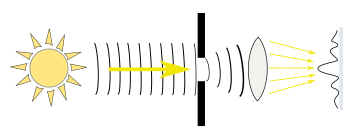Fig. 10. Normal single slit diffraction setup. A single color source provides light (a wave) which propagates over to an opaque barrier that has a slit in it to allow a narrow beam of light to pass. On the far side of the slit this light spreads out (diffracts) and at a large distance (or with the help of a lens to allow shorter distances) we see a standard wave Fraunhofer interference diffraction pattern on a screen. Double slit diffraction uses two slits in the opaque barrier and produces a little different diffraction pattern. A diffraction grating uses many slits and produces yet a third diffraction pattern. For computer simulation all the stuff on the left side of the barrier and the barrier itself can be replaced by a linear array of emitters. We will explore this further below.

Next we will algebraically derive these diffraction patterns for an array of monopole emitters. Since the wave of a monopole is made up of Hankel functions and Fraunhofer diffraction is all about the wave field at large distances, we look at the behavior of Hankel functions for very large arguments. It is also important for the approximation that the patterns be relatively close to the x axis.

At very large arguments (z >> |α2+ ¼| ) the Hankel functions can be approximated by an asymptotic sinusoid:

Using (4) the asymptotic wave field of a monopole emitter becomes:

where

is the distance from an emitter at (x,y) = (0,y1) to a point (x2,y2) on the observation screen.

The normal way to calculate Fraunhofer diffraction is to imagine a continuum of emitters located on the y axis and extending from y = −a/2 to y = +a/2. The parameter a is the width of the slit (or opening) in the opaque barrier. We set up an integral to sum up the waves from these emitters.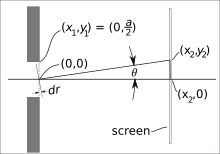Fig. 11. Geometric setup. Waves coming in from the left pass through the slit and some are diffracted up at angle θ to a point (x2,y2) on the observation screen. The center of the slit is considered the origin (0,0). The vertical distance that a diffracting point is above or below the origin inside the slit is denoted by y1.

To get to a simple mathematical equation, we need to approximate the r in (6) and (7) consistent with the screen being at a large distance from the slit, large compared with the wavelength of the waves. This calculation involves replacing the r in the exponent in (7) with an average distance r0 plus small dr that varies with the angle, i.e. dr = −y1 sinθ where θ is the angle between the line from y1 to y2 and the x axis as shown in Fig. 11:

The r in the denominator of the square root in (7) is considered slowly varying and replaced by r0 ≅ x2. This is in contrast with the r in the exp() function which, because of it sinusoidal nature, small changes in r cause large changes in the result of the exp() operation.

The C in (8) stands for the lumped together complex constants which do not play a role in the amplitude pattern of the waves striking the screen.

An alternative way to derive (8) is to approximate (6) with the first two terms of a Taylor series expansion.

Fraunhofer diffration simulation - various methodsFig. 12. Fraunhofer diffraction as calculated by various methods. The various curves are offset vertically a little bit from each other to make them more readable.

The bottom three curves are calculated via the same simulation as used by the 2D mappings in Figs. 7, 8 and 9 above, but calculated at a distance of x = 500. These three curves are virtually identical which indicates that in the Fraunhofer diffration limit, the exact nature of the emitter is not important.

The top three curves are calculated via the Fraunhofer approximation formula, the purple curve letting the computer sum the approximate waveforms of (5) at the designated x and y points. The turquoise and light brown curves used Equation (8) with the turquoise one using the sinθ version and the light brown curve using the final expression. Because the diffraction angle θ at the edges of the graph is arctan(400/500) = 39 degrees the approximation that sinx ≅ x is not very good at the edges.

The x axis on the graph indicates y2 values at x2 = 500. Plotted vertically are the values of the complex magnitude of the waves at the various y2's as indicated and x2 = 500.

The two sets of curves differ from each other mostly in their tails, the regions to the left and right of the central peak. This indicates that the Fraunhofer approximation has increasing errors at larger angles.Fig. 13a. Field strengths were measured at the locatioins shown in green, very close to the linear array of emitters (shown in red).

### Beam strength very close to an emitter array

For the next set of simulations it is important to know the ratio between the strength of the emitters in a linear array and the amplitude of waves in the beam once the waves from the various emitters have more or less merged. Below are graphs of the amplitude of the partially "merged" beams for emitter strengths of 1.0 .

Amplitude versus y for monopole emitters Amplitude versus y for dipole emittersAmplitude versus y for directional emittersFig. 13b. Using a computer simulation in Octave the amplitude of waves very close to the array of emitters are plotted. The three graphs are for the three types of emitters (monopole, dipole and directed) as a function of y as shown in Fig 13a.

In all, 21 emitters were used in a "slit" width of 10. The three curves in each graph represent the fields at 0.5, 1.0 and 1.5 away from the emitters. All curves were normalized by multiplying by a factor of (π/2)×A/emitterDensity where the emitterDensity = N/a = 21/10  in this case. This normalization allowed the average amplitude to equal 1 for many different settings of the slit width a and the number of emitters N.

The three curves on each graph are offset vertically from each other a little to allow better viewing.

### Reflection via emitters

To illustration an application of arrays of emitters, we next simulate a mirror using a linear array of monopole emitters. The strengths of the emitters in the mirror are set to cancel out the incident wavefront using the (π/2)×A/emitterDensity factor mentioned in the caption for Fig. 13 above.

 Fig. 14. A wave is produced by a linear array of monopole emitters located at the origin and propagates to a second linear array of monopole emitters which is set at an angle. The amplitude of each reflector emitter is set to cancel the initial wavefront in the space beyond the mirror. In the process a reflected beam is automatically produced. The left most image is a snap shot, frozen in time, while the right image is a movie. The movie uses a smaller wavelength than the snap shot.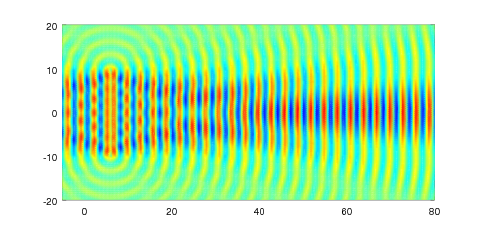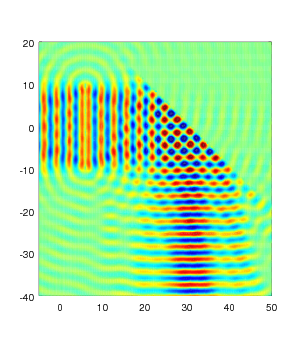Fig. 15. Comparing the waves without and with the reflector. The reflector is made of a series of monopole emitters set to cancel the incident wave in the space beyond the mirror. In the process a reflected beam is automatically made. In the left most image the interference details of near field waves are very evident.

### Resonant cavities via emitters

One form of resonators is a resonant cavity. This type of resonator consists of a chamber with walls that reflect all waves inside the chamber and keep them bottled up. The waves can be optical, microwave, acoustical, water waves, etc and the chamber walls made of material suitable for the wave type. The best cavity resonators have very low loss so that the waves will reflect on themselves many, many times. If a driving source of the waves is inserted into the chamber and its frequency is adjusted just so, very large standing waves will develop in time. The frequencies at which this occurs are called the resonant frequencies.

Above we have just explored the use of a linear array of emitters to form a reflector of waves. We will next investigate the use of such an array to form a cavity resonator.

Above we set the strength of emission of each wall emitter so that it canceled the waves on the far side of the mirror. We set the strength of emission based on the waves present from all other sources. (These are the waves we wish to cancel on the far side.) We should be able to repeat this method here.

Instead we decided to change the method a little. We added a separate array of sensors located a distance outside the cavity and mathematically requiring that the complex amplitude of each wall emitter be adjusted so that the total amplitude detected at each of these external sensors was zero. This separated the functions of detecting and emitting.

#### circular cavity

Below we see the results of an Octave simulation using a circular array of monopole emitters forming a circular cavity as shown in Fig. 16. There is a single active monopole emitter at the center of the circle to drive the resonance. There is also a cicular array of sensors outside the walls.

We want the wall emitters to broadcast a wave that cancels the wave from the central driver and all other wall emitters at the sensors' locations. We can express this mathematically as:

where

1. Φjthsensor is the wave at the jth sensor's location due to waves sent out by the driver and by all the cavity wall emitters.
2. rdrive - jthsensor is the distance between the driver and jth sensor. We will refer to this distance as r0,j.
3. rithwall - jthsensor is the distance between the ith wall emitter and jth sensor. We will refer to this distance as ri,j.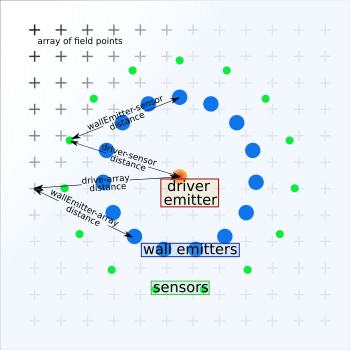Fir. 16. Emitters, sensors and distances used to model a resonant cavity using emitters. The orange dot in the center is the driver that sends out waves to drive the resonance. The blue dots represent the wall emitters acting as a barrier to the waves from the central driver by creating counter waves to cancel the driver's waves outside the cavity. The green dots represent sensors that detect waves and are used to mathematically set the correct amplitude of the counter waves to properly cancel the driver's waves and the waves from the other wall emitters. The array of field point are the points over which the total field is calculated to create the 2D color display of the waves everywhere.

In Fig. 16 we show these points and distances.

In (8), the Ai's, the complex amplitudes of the wall emitters, are the unknowns while everything else are the knowns.

We can write (8) as a linear matrix equation:

where

In standard matrix notation we write (9) as:

M A = -S      ,      (11)

where A and S are vectors and M is a matrix.

In Octave, we first calculate S and M based on the geometry of our setup. We then use the standard linear matrix equation solver, linsolve, to solve for A. Once we know A we know the strengths of all emitters in the setup and we can calculate the field anywhere to make our 2D false color maps.

where p is the point at which you want to know the wave fields. In figure 16 we show these query points as an "array of field points" which we will use to make a 2D color map of the fields.

Resonant cavity simulation via emitters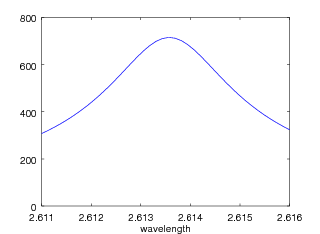Figs. 17a and 17b. Cavity made of 15 emitters. The left image is a 2D false color mapping of the magnitude of waves for the first resonance, the (0,1) mode.

The right image is a graph of the amplitude near the center versus the frequency of the driver (which affects κ, the wavenumber). We see the standard resonance curve. This resonance has a Q of about 1200. (For more on Q's of resonators see this reference and other links at the beginning of that reference.)

Use of more wall emitters will result in much higher Q's.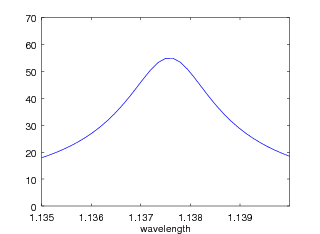Figs. 18a, 18b and 18c. The first two images are similar to the above images except they are for the second mode. Also we have plotted the real part of the complex wave (which goes positive and negative) instead of its magnitude. Zero field is light green as indicated by the colorbar. While the previous mode has only a central peak, this mode has the central peak plus an outer ring (blue). (For more on modes of a circular resonator see this reference.)

The last image shows the real part of the complex wave versus the radius (or x when constrained to a radial line). We see with Dirichlet boundary conditions, the field peaks at r = 0 goes through zero at r = 0.42, peaks in the negative at r = 0.7 and returns to zero at r = 1 which is the cavity wall. The field is zero outside the cavity. The precise field shown at the cavity wall depends on the exact path we chose through the array of emitters there. We try to pass cleanly through a space between the emitters and avoid passing too close to an emitter whose fields can be very strong.

Mathematically we expect circularly symmetric modes at wavelengths of 2.6 and 1.14 times the cavity resonance radius. The radius of our cavity is 1.0 . This resonance has a Q of about 750. This resonance has a lower Q than that above because it is of higher frequency and thus shorter wavelength which tends to "slip" through our picket fence of emitters a little better.

We might point out that the above setup is very symmetric and could be solved without matrix solving methods. On the other hand, the method used above is general and directly applicable to non-symmetrical and oddly shaped cavity resonators (such as the L-shaped resonator discussed below). Treating such resonators involves a somewhat more complicated routine to specify the coordinates of the cavity wall emitter x,y locations.

modeling a rotating wave resonator

In an earlier posting we have discussed rotating wave fields in depth.

In fig. 19 we show use of the above methods to make an animation of a rotating mode in our cavity.

 Fig. 19. Video clip of the fields in the (2,1) rotating mode modeled in Octave using a circular array of emitters for the cavity wall. Two driver monopole emitters were used, one on the x-axis at (x,y)=(0.5,0) and one above it at (x,y)=(3.5,3.5), both positioned to be near the maximum field regions of their related standing wave modes. Fields from the second one were multiplied by the imaginary constant i, to cause a 90 degree temporal phase shift between the two standing wave modes. Fields of the two modes were then added together on a point-by-point basis and used to make the color video shown.

### L-shaped resonator

As an example of applying these emitter methods to other shaped resonators we next model an L-shaped resonator. The method is the same as above except that calculation of the resonator wall emitter locations is more burdensome and the calculation of the sampling points is even more complicated.

In order to avoid spurious resonances, each of the exterior sampling points needs to be closest to its own special wall emitter. This requirement entails a somewhat "messy" specifying of the sampler locations. Enough so that while we wrote the code for the wall emitter placement quite generally for a polygon resonator, we custom fit the sample point locations to our particular L-resonator at hand.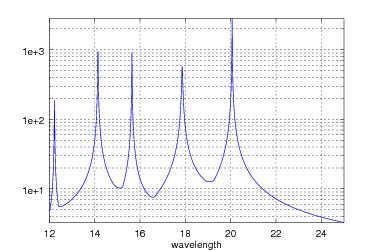Fig. 20. Layout for the L-shaped cavity and the response spectra for this cavity obtained via the emitter methods discussed above. The wall emitters are shown as green x's, the sampling points as red dots and the exciting source as a turquoise asterisk.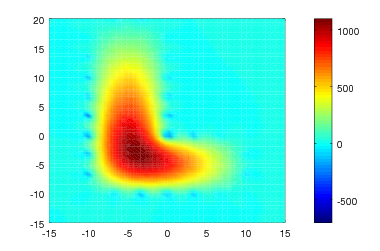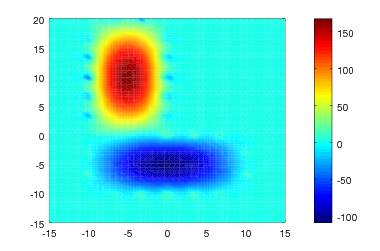Fig. 21. First three modes of the L-shaped resonator. As shown in Fig. 19 above these occur at wavelengths of roughly 20, 18, and 16 (more exactly at 20.065, 17.862 and 15.643). The right most peak belongs to the wavelength of 20. Note that in all cases one half wavelength is less than the cavity width, as might be expected. This method pretty much ensures that the field outside the resonator is zero; however, it doesn't ensure that the fields will be zero right at the site of the wall emitters. In fact in the field mappings we see that there are non-zero fields at the sites of many of the wall emitters.

### Resonator with Neumann boundary conditionsFig. 22. Angles involved with orienting dipole emitters. This is basically a repeat of a section of Fig. 16 with the blue dots representing the cavity wall emitters (dipole emitters in this case). The driver emitter is at the center of the circular cavity. We have shown a representation of the dipole fields emitted by one of the wall emitters.

Neumann boundary conditions can be simulated with emitters by substituting dipole wall emitters in place of the monopole emitters used above for Dirichlet boundaries. The dipole emitters are a little more complicated to use because their field strength varies with direction requiring each of them to be properly oriented. The correct orientation is with the maximum emission being perpendicular to the cavity wall so that their fields add to the fields inside the cavity and subtract from the fields outside.

Suppose we have a dipole that is oriented horizontally. The field from this, radiating at ϕ2 relative to the horizontal, is given by:

which we repeat from (2) above. But our dipoles are not all oriented horizontally. Instead they are arranged around the circular cavity walls, everywhere perpendicular to these walls, as shown in Fig. 22. So what is the field for a dipole in the wall of our circular resonator as a function of its position in the resonator? That is to say, what is ϕ2 for (13) as a function of the polar angle ϕ1 that an emitter happens to be located at? By examining Fig. 22 we see that these angles are related by:

where ϕ3 is the angle relative to the horizontal for which we want the emission.

Fig. 23 shows the results of an Octave simulation using an array of dipoles in place of the cavity walls.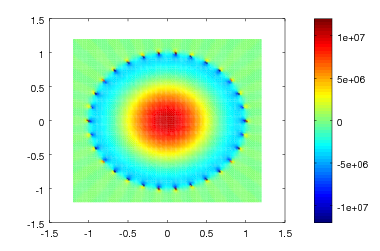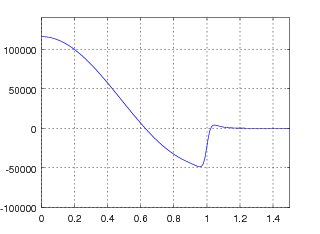Fig. 23. The (0,1) circularly symmetric mode of a circular cavity with Neumann boundary conditions. This simulation was made using 30 dipole emitters to simulate the cavity wall. The Q of this simulated resonance was over a million. Fig. 24. Graph of the field strength at one instant in time as a function of radius. We see at the cavity wall, at x = 1, the field abruptly changes from a healthy value to zero. We also see that the derivative of the field with respect to x is approximately zero at this point.

### Overview

Let's reflect on the work shown above to model reflectors. We have used two methods. To make the reflectors shown in Figs. 14 and 15 we required that the reflector emitters react to the wave fields they sense at their location and zero this out. This method could be viewed as how atoms in real wave reflectors respond to incident waves and is therefore very natural to use.

This method can be extended by replacing the monopole emitters with dipole emitters oriented perpendicular to the surface to allow modeling Neumann boundary conditions (most of the above examples assumed Dirichlet boundary conditions.) Use of directional emitters, also oriented perpendicular to the surface, would allow modeling wave absorbing surfaces.

The question that we worked on in Fig. 13 but did not totally resolve is:

"exactly what field should or would an elementary emitter or atom emit for a given applied field?"

Another way to state this question is: "how stong should a wall emitter's amplitude A be so that its emitted waves merge with that of nearby emitters to cancel the total wave on the far side of a mirror?". This brings up the (π/2)×A/emitterDensity factor we mentioned in Fig. 13 above. Does this factor hold for corners in a segmented reflector as in the L-shaped resonator above? Does it hold for curved reflectors such as the walls of the circular resonator in Figs. 16 through 19?

Method 2 bypasses that question by using external sensors. It works nicely in a case where we know what the field should be at the sensor locations, as in zero outside a resonator.

If we answered the above question, we could use the first method in situations where we do not have regions of zero field or known field strength and wish to find the field everywhere. It would also open up the emitter method for use where dielectric-like materials are present. In this case we would model a dielectric as an array of emitters set to react less vigorously to the applied field as would an emitter in a conductor.

### The diffraction question

Question of the exact nature of diffraction often comes up.
• If we have a narrow, well-collimated beam traversing an otherwise empty wave medium, will the beam diffract? Exactly when and where will this occur?
• Some spreading will take place from the instant the beam emerges from the collimating structure.
• From the Huygens-Kirchhoff diffraction formula we can pick the beam anywhere and computationally let it diffract at that point with a (1+cosθ) dependence. We might think that this would lead to immediate destructive spreading out of the beam. However a careful considering of the H-K formula shows that the beam with a reasonable width will resist spreading out, except for an insignificant amount. When we add all the wavelets from the whole beam width, the diffracting part is almost entirely canceled out.
• Waves cannot escape their tendency to diffract and spread out, however the amount of wave energy that goes to really odd unintuitive trajectories is extremely small.
• It would be interesting to do a real time double slit modeling using the H-K formula with Hankel functions. Launch a short pulse of waves and watch them do all sorts of unintuitive things as a movie or series of stills. To see this, the log of the amplitude will need to be displayed in a 2D false color map. Might make an entertaining movie to show at a conference.